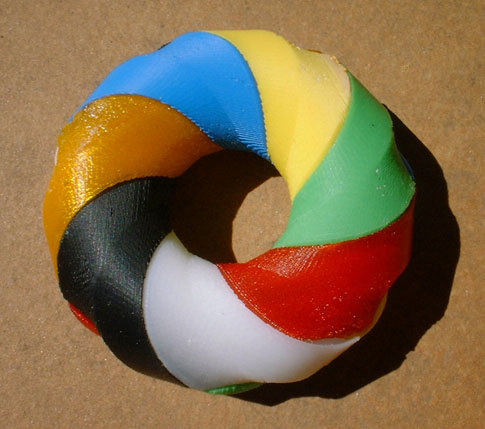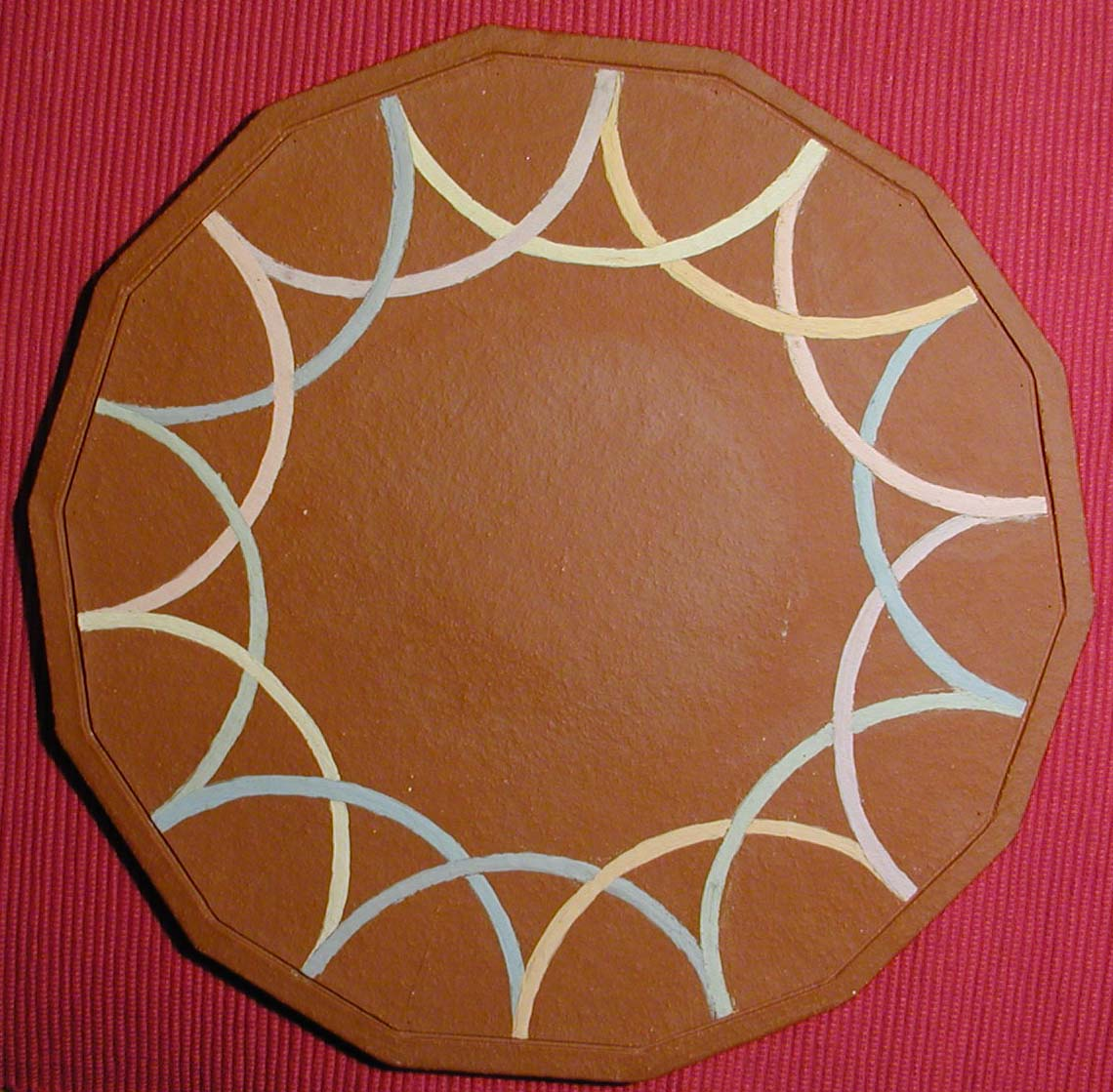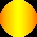ON TORI OF CSÁSZÁR AND SZILASSI AND MORE

JÜRGEN BOKOWSKI

 Name: Bokowski J., Mathematician (b. Elbing, Germany, 1943). Address: Department of Mathematics, Darmstadt University of Technology,  Schloßgartenstr. 7, D-64289 Darmstadt, Germany.  E-mail: juergen@bokowski.de Fields of interest: Convex geometry, discrete geometry.

 Abstract: Császárs polyhedral torus (1949) and Szilassis polyhedral torus (1977) are celebrated dual polyhedra. The combinatorics of Császárs torus was mentioned by Möbius in 1861 the combinatorics of Szilassis torus is known as Heawoods map. Császárs polyhedron has, like a tetrahedron, no diagonals. Can we find other polyhedra without diagonals? There are infinitely many candidates: triangular complete graph embeddings on surfaces. However no such additional polyhedral realization is known. Bokowski and Guedes de Oliveira have shown a first non-realizable example in 2000. We provide a Haskell code proof showing that we have e.g. a 4-dimensional convex polyhedron with 8 vertices without diagonals. We mention spatial polyhedral pinched spheres without diagonals.

1 COMBINATORICS OF TORI OF CSÁSZÁR AND SZILASSI

From a square with diagonal (1,3) we lift vertices 4 and 7. Insert 4 triangles as shown. Points 5 and 6 lie higher than all previous ones. Add two additional triangles. An apex above the midpoint of the original square together with a roof structure of additional 6 triangles tells us the shape of the torus of Császár. Szilassis torus is shown on the right with its seven non-convex facets. Both polyhedra have a geometrical symmetry of order 2. This is optimal, see Bokowski and Eggert 1991 and Bokowski and Schewe 2004.Figure 1: Császár.s torus (left) and Szilassis torus (right).

Let us study its combinatorial symmetries. Look at the ring surface depicted as our first pottery model. Six curved edges start at each vertex reaching all the remaining vertices. This complete graph embedding published Möbius in 1861.Figure 2: From left to right: Möbiuss torus, Heawoods map, Curve arrangement.

We place a vertex in each curved triangle and we connect vertices when the corresponding triangles have a common edge to obtain Heawoods map, see a nice model of Carlo Séquin in the middle. Both combinatorial objects are regular maps.Figure 3: Cuve arrangement on a surface of genus 3.

The related pottery object of the author on the right shows seven simple closed curves on a genus 3 surface. The two parts of each curve with a common colour have to be connected. We identify seven pairs of edges of the regular 14-gon when the same pair of curves meet and in such a way that the curves cross. Each pair of curves has precisely one intersection point. The next pottery model shows the curves on the surface. The surface hides the symmetry, however, it reveals the additional combinatorial symmetry of order 3. The combinatorial symmetry group is known to be of order 42.

We have seven points in Möbiuss torus, seven cells in Heawoods map, seven curves on the genus 3 surface. Any two points are joint by an edge in case of Möbiuss torus, any two cells have an edge in common in Heawoods map and any two curves have a common intersection point. The incidence structure in all three cases is identical. This is indeed the starting point for a transition from graph embeddings on surfaces to curve arrangements on surfaces. The case of simple closed curves in the projective plane lead to the theory of oriented matroids, see Björner et al. 1993 and Bokowski, 2005.

2 ON OTHER SPATIAL POLYHEDRA WITHOUT DIAGONALS

There are other triangular complete graph embeddings in great profusion. However, not a single example out of infinitely many of them has been realized as a polyhedron.Figure 4: Complete graph with 12 vertices on a surface of genus 3.

Imagine that the next smallest case looks already somehow like the above K12 embedding on a genus 6 surface with 44 triangles. Bokowski and Guedes de Oliveira have shown that in this particular example a search for coordinates such that the polyhedron has no self-intersections will be in vain. A corresponding polyhedral realization can simply not exist. See additional comments in Bokowski 2001.

3 4-DIMENSIONAL POLYHEDRON WITHOUT DIAGONALS

In dimension four there are convex polyhedra without diagonals for any given number of vertices. A Haskell code (functional programming) exemplifies the case with 8 vertices.

 altOM::Int->Int->[([Int],Int)] -- chirotope of cyclic 4-polytope altOM r n=zip(tuples r n)(replicate(length(tuples r n))1) simplicialFacetsChi::[([Int],Int)]->[[Int]] simplicialFacetsChi chi =[p|p<-tuples(r-1)n,(and[norm(p++[x], 1)`elem`chi|x<-[1..n]\\p]) ||(and[norm(p++[x],-1)`elem`chi|x<-[1..n]\\p])] where r=length(fst(head chi)); n=maximum(concat (map fst chi)) norm::([Int],Int)->([Int],Int) norm (tuple@(h:rest),sign)  |rest ==[]=([h],sign)|h==minimum tuple=([h]++fst next,snd next) |odd(length rest)=norm(rest++[h],-sign) |otherwise=norm(rest++[h], sign) where next=norm(rest,sign)  tuples::Int->Int->[[Int]]; tuples 0 n = [[]]  tuples r n = tuplesL r [1..n] tuplesL::Int->[Int]->[[Int]]  tuplesL r list@(x:xs)  |length list

4 SPATIAL PINCHED SPHERES WITHOUT DIAGONALS

There are spatial polyhedral realizations of pinched spheres that have no diagonals, see Altshuler, Bokowski, Schuchert (1993) and (1994).

References

Altshuler, A., Bokowski, J., and Schuchert, P. (1993) Sphere systems and neighborly spatial polyhedra with 10 vertices, Proceedings of the First International Conference on Stochastic Geometry, Convex Bodies and Empirical Measures, Palermo, Italy, Supplemento ai rendiconti del Circolo matematico di Palermo, Ser. II, No. 35, 1994.

Altshuler, A., Bokowski, J., and Schuchert, P. (1994) Spatial polyhedra without diagonals, Israel J.Math., 88, 373-396.
Altshuler, A., Bokowski, J., and Schuchert, P. (1996) Neighborly 2-manifolds with 12 vertices, J. Comb. Theory, Series A, 75, No. 1, 148-162.
Bokowski, J. (2001) Pottery models and complete graphs on surfaces, Symmetry: Art and Science,Proceedings of the Sydney Congress of ISIS-Symmetry.
Bokowski, J. (2005) Computational Oriented Matroids, Cambridge University Press, to appear.
Bokowski, J. and Eggert, A. (1991) All realizations of Möbius torus with 7 vertices, Structural Topology, No. 17, 59-78.
Bokowski, J. and Guedes de Oliveira, A. (2000) On the generation of oriented matroids, Discrete and Computational Geometry, 24, 197-208.
Bokowski, J. and Pisanski, T. (2001) Oriented matroids and complete graph embeddings on surfaces,J. Comb. Theory, Series A, submitted.
Björner, A., Las Vergnas, M., Sturmfels, B., White, N.,Ziegler, G. (1993) Oriented Matroids, Cambridge:Cambridge University Press
Czászár, Á.(1949) A polyhedron without diagonals, Acta Sci. Math. Universitatis Szegediensis, 13, 140-142.
Lutz, F. (2002) Czászárs torus, Electronic geometry model, No. 2001.02,069.
Ringel, G. (1974) Map Color Theorem, Springer, Berlin, Heidelberg, New York.
Szilassi, L. (1977) Egy poliéder, melynek bármely két lapja szomszédos, Juhász Gyula Tanárképz?F?iskola Tudományos Közleményei, 2, Szeged, 130-139. (In Hungarian.)
Szilassi, L. (1986) Regular toroids, Structural Topolgy, No. 13, 69-80.

Additional note: Möbius's torus and its dual, the Heawood map are no regular maps in the strongest sense.